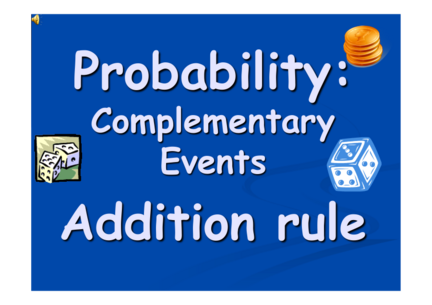# Probability Complementary Events: Addition RuleContributed by:This pdf covers the following topics:-
Complementary Events
Disjoint Events
Non-Disjoint Events
1. Complementary
Events
2. Complementary Events
the events of one outcome happening
and that outcomes not happening
are complementary (opposite)
( not E is contrary event to E )
E Not E
For example : you pick up a card from a deck
E: P(Heart)= ¼
Not E:  P(not Heart)= ¾
3. Complementary Events
THE SUM of the of the probabilities of
complementary events is 1.
Not E
from which I get:
: Probability of the contrary event Not E is:
“1 minus the Probability of the event E”
Not E
4. Complementary Events
You pick up a card from deck of 52 cards.
Which is the probability of picking a figures?
12
P( figures) =
52
Which is the probability of not picking a
figures ?
12 52 − 12 40
P(NotFigures) = 1− = =
52 52 52
5. Complementary Events
1) The probability that it will rain tomorrow is 0.4 .
What is the probability it does not rain ?
P(not Rain) = 1-0.4 = 0.6
2) Tossing 2 coins,which is the probability of:
a) never getting Tail ?
b) getting at least once Tail?
(TT or HT or TH )
P(at Least Once T)=1-P(never T)=1-1/4= 3/4
PROBABILITY OF
A OR B
7. 1) Disjoint Events
There are two situations
2) NOT Disjoint Events
Disjoint Events ?
Two events are Disjoint ( Mutually Exclusive ) if they
can't happen at the same time
 Turning left and turning right are Mutually Exclusive (you can't do
both at the same time)
 Cards: Kings and Aces are disjoint
What is Not Disjoint ( not Mutually Exclusive ) ?
 Turning left and scratching your head can happen at the same time
 Cards: Kings and Hearts, because we can have a King of Hearts!
8. 1) DISJOINT Events (Mutually Exclusive )
A single card is chosen at random from a
standard deck of 52 playing cards.
What is the probability of choosing an Ace
or a King?
P(ACE or KING ) = P(Ace) + P(King)
= 4/52 + 4/52 = 8/52
9. 1) DISJOINT events
A
for DISJOINT
Events:
When two events A and B are disjoint, the
probability that A or B will occur is:
the SUM of the Probability of each Event.
P(A or B) = P(A) + P(B)
10. 2) NOT DISJOINT ( NOT Mutually Exclusive)
example
A single card is chosen at random from a
standard deck of 52 playing cards.
What is the probability
of choosing
an Heart or a King?
P(H or K) = P(H) + P(K) - P(both)
=13/52 + 4/52 –1/52 = 16/52
11. A and B = intersection
1) NOT DISJOINT
for NOT disjoint A or B = union
Events
When two events A and B are NOT DISJOINT,
the probability that A or B will occur is :
the SUM of the probability of each event,
MINUS the probability of the overlap.
both
P(A or B) = P(A) + P(B) - P(A and B)
U union ∩ Intersection
DISJOINT EVENTS NOT DISJOINT EVENTS
Mutually Exclusive Not Mutually Exclusive
A and B together is impossible:
P(A and B) = 0 A and B together is possible !
P(A or B) = P(A) + P(B) P(A or B) = P(A) + P(B) − P(A and B)
13. TRY IT YOURSELF
TEST
1: A single card is chosen at random from a
standard deck of 52 playing cards. What is the
probability of choosing an Ace or a figure?
2: A single card is chosen at random from a
standard deck of 52 playing cards. What is the
probability of choosing an Ace or Red Card?
3: You are going to roll two dice. Find:
P(sum that is even or sum that is a multiple of 3).
14. 1: A single card is chosen at random from a
standard deck of 52 playing cards. What is the
probability of choosing an Ace or a figure?
Figures
P(Ace)=4/52 Aces
 These events are mutually exclusive ( disjoint)
since they cannot occur at the same time.
P(A or B) = P(A) + P(B)
U union
P(Ace OR Figure) = 4/52+12/52 = 16/52
15. 2. A single card is chosen at random from a
standard deck of 52 playing cards. What is the
probability of choosing an Ace or Red Card?
Aces Red cards
P(Red card)=26/52
 These events are NOT disjoint since they have
some overlap ( favorable outcomes in common )
P(A or B) = P(A) + P(B) - P(A and B)
U union ∩ Intersection
P(Ace OR Red Card) = 4/52+26/52-2/52 = 28/52